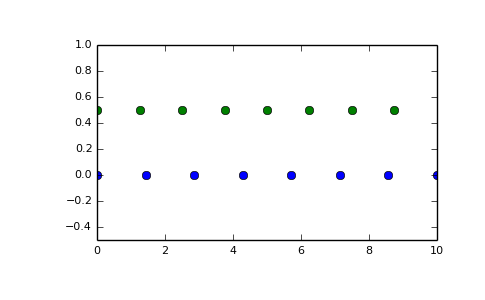# numpy.linspace¶

numpy.linspace(start, stop, num=50, endpoint=True, retstep=False, dtype=None)[source]

Return evenly spaced numbers over a specified interval.

Returns num evenly spaced samples, calculated over the interval [start, stop].

The endpoint of the interval can optionally be excluded.

Parameters: start : scalar The starting value of the sequence. stop : scalar The end value of the sequence, unless endpoint is set to False. In that case, the sequence consists of all but the last of num + 1 evenly spaced samples, so that stop is excluded. Note that the step size changes when endpoint is False. num : int, optional Number of samples to generate. Default is 50. Must be non-negative. endpoint : bool, optional If True, stop is the last sample. Otherwise, it is not included. Default is True. retstep : bool, optional If True, return (samples, step), where step is the spacing between samples. dtype : dtype, optional The type of the output array. If dtype is not given, infer the data type from the other input arguments. New in version 1.9.0. samples : ndarray There are num equally spaced samples in the closed interval [start, stop] or the half-open interval [start, stop) (depending on whether endpoint is True or False). step : float Only returned if retstep is True Size of spacing between samples.

arange
Similar to linspace, but uses a step size (instead of the number of samples).
logspace
Samples uniformly distributed in log space.

Examples

```>>> np.linspace(2.0, 3.0, num=5)
array([ 2.  ,  2.25,  2.5 ,  2.75,  3.  ])
>>> np.linspace(2.0, 3.0, num=5, endpoint=False)
array([ 2. ,  2.2,  2.4,  2.6,  2.8])
>>> np.linspace(2.0, 3.0, num=5, retstep=True)
(array([ 2.  ,  2.25,  2.5 ,  2.75,  3.  ]), 0.25)
```

Graphical illustration:

```>>> import matplotlib.pyplot as plt
>>> N = 8
>>> y = np.zeros(N)
>>> x1 = np.linspace(0, 10, N, endpoint=True)
>>> x2 = np.linspace(0, 10, N, endpoint=False)
>>> plt.plot(x1, y, 'o')
[<matplotlib.lines.Line2D object at 0x...>]
>>> plt.plot(x2, y + 0.5, 'o')
[<matplotlib.lines.Line2D object at 0x...>]
>>> plt.ylim([-0.5, 1])
(-0.5, 1)
>>> plt.show()
```numpy.arange

numpy.logspace